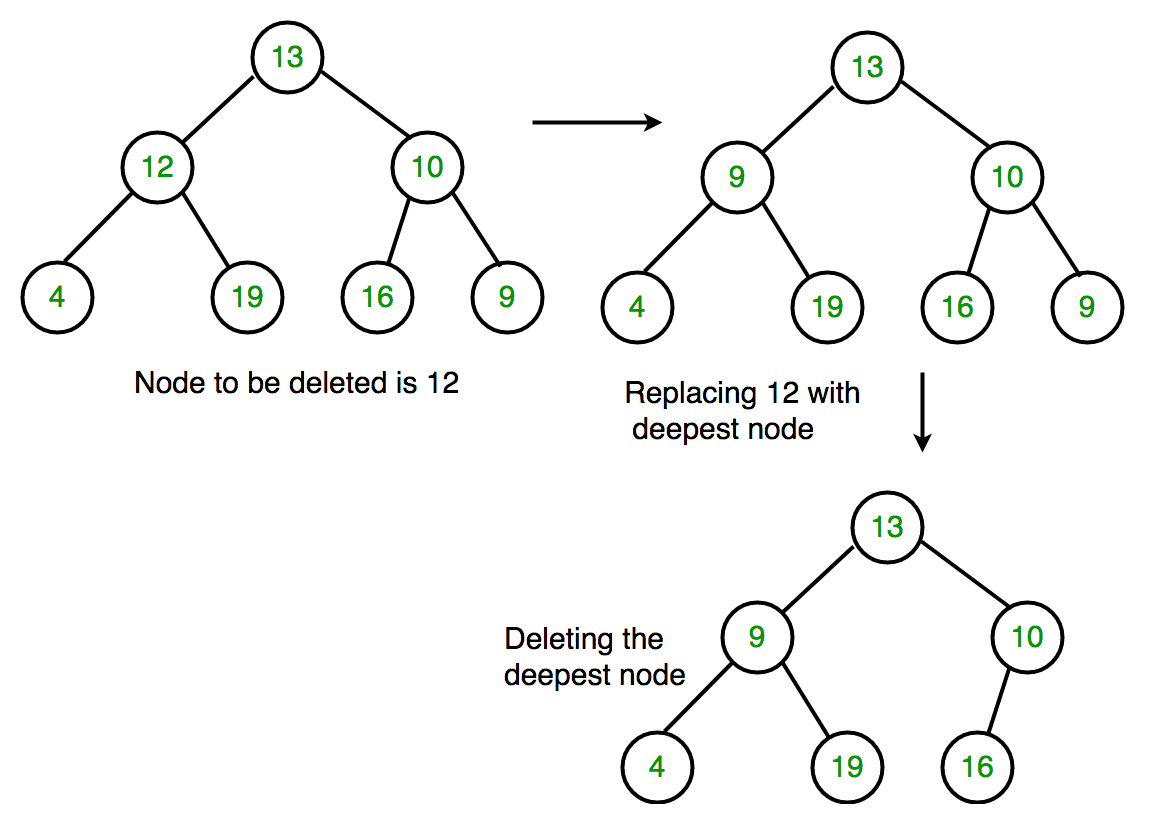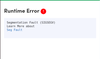Related Articles

# Deletion in a Binary Tree

• Difficulty Level : Medium
• Last Updated : 17 Sep, 2021

Given a binary tree, delete a node from it by making sure that tree shrinks from the bottom (i.e. the deleted node is replaced by the bottom-most and rightmost node). This is different from BST deletion. Here we do not have any order among elements, so we replace with the last element.

Examples :

```Delete 10 in below tree
10
/    \
20     30
Output :
30
/
20

Delete 20 in below tree
10
/    \
20     30
\
40
Output :
10
/   \
40    30   ```

Algorithm
1. Starting at the root, find the deepest and rightmost node in binary tree and node which we want to delete.
2. Replace the deepest rightmost node’s data with the node to be deleted.
3. Then delete the deepest rightmost node.## C++

 `// C++ program to delete element in binary tree``#include ``using` `namespace` `std;` `/* A binary tree node has key, pointer to left``child and a pointer to right child */``struct` `Node {``    ``int` `key;``    ``struct` `Node *left, *right;``};` `/* function to create a new node of tree and``return pointer */``struct` `Node* newNode(``int` `key)``{``    ``struct` `Node* temp = ``new` `Node;``    ``temp->key = key;``    ``temp->left = temp->right = NULL;``    ``return` `temp;``};` `/* Inorder traversal of a binary tree*/``void` `inorder(``struct` `Node* temp)``{``    ``if` `(!temp)``        ``return``;``    ``inorder(temp->left);``    ``cout << temp->key << ``" "``;``    ``inorder(temp->right);``}` `/* function to delete the given deepest node``(d_node) in binary tree */``void` `deletDeepest(``struct` `Node* root,``                  ``struct` `Node* d_node)``{``    ``queue<``struct` `Node*> q;``    ``q.push(root);` `    ``// Do level order traversal until last node``    ``struct` `Node* temp;``    ``while` `(!q.empty()) {``        ``temp = q.front();``        ``q.pop();``        ``if` `(temp == d_node) {``            ``temp = NULL;``            ``delete` `(d_node);``            ``return``;``        ``}``        ``if` `(temp->right) {``            ``if` `(temp->right == d_node) {``                ``temp->right = NULL;``                ``delete` `(d_node);``                ``return``;``            ``}``            ``else``                ``q.push(temp->right);``        ``}` `        ``if` `(temp->left) {``            ``if` `(temp->left == d_node) {``                ``temp->left = NULL;``                ``delete` `(d_node);``                ``return``;``            ``}``            ``else``                ``q.push(temp->left);``        ``}``    ``}``}` `/* function to delete element in binary tree */``Node* deletion(``struct` `Node* root, ``int` `key)``{``    ``if` `(root == NULL)``        ``return` `NULL;` `    ``if` `(root->left == NULL && root->right == NULL) {``        ``if` `(root->key == key)``            ``return` `NULL;``        ``else``            ``return` `root;``    ``}` `    ``queue<``struct` `Node*> q;``    ``q.push(root);` `    ``struct` `Node* temp;``    ``struct` `Node* key_node = NULL;` `    ``// Do level order traversal to find deepest``    ``// node(temp) and node to be deleted (key_node)``    ``while` `(!q.empty()) {``        ``temp = q.front();``        ``q.pop();` `        ``if` `(temp->key == key)``            ``key_node = temp;` `        ``if` `(temp->left)``            ``q.push(temp->left);` `        ``if` `(temp->right)``            ``q.push(temp->right);``    ``}` `    ``if` `(key_node != NULL) {``        ``int` `x = temp->key;``        ``deletDeepest(root, temp);``        ``key_node->key = x;``    ``}``    ``return` `root;``}` `// Driver code``int` `main()``{``    ``struct` `Node* root = newNode(10);``    ``root->left = newNode(11);``    ``root->left->left = newNode(7);``    ``root->left->right = newNode(12);``    ``root->right = newNode(9);``    ``root->right->left = newNode(15);``    ``root->right->right = newNode(8);` `    ``cout << ``"Inorder traversal before deletion : "``;``    ``inorder(root);` `    ``int` `key = 11;``    ``root = deletion(root, key);` `    ``cout << endl;``    ``cout << ``"Inorder traversal after deletion : "``;``    ``inorder(root);` `    ``return` `0;``}`

## Java

 `// Java program to delete element``// in binary tree``import` `java.util.LinkedList;``import` `java.util.Queue;` `class` `GFG{``    ` `// A binary tree node has key, pointer to``// left child and a pointer to right child``static` `class` `Node``{``    ``int` `key;``    ``Node left, right;``    ` `    ``// Constructor``    ``Node(``int` `key)``    ``{``        ``this``.key = key;``        ``left = ``null``;``        ``right = ``null``;``    ``}``}` `static` `Node root;``static` `Node temp = root;` `// Inorder traversal of a binary tree``static` `void` `inorder(Node temp)``{``    ``if` `(temp == ``null``)``        ``return``;` `    ``inorder(temp.left);``    ``System.out.print(temp.key + ``" "``);``    ``inorder(temp.right);``}` `// Function to delete deepest``// element in binary tree``static` `void` `deleteDeepest(Node root,``                          ``Node delNode)``{``    ``Queue q = ``new` `LinkedList();``    ``q.add(root);``    ` `    ``Node temp = ``null``;``    ` `    ``// Do level order traversal until last node``    ``while` `(!q.isEmpty())``    ``{``        ``temp = q.peek();``        ``q.remove();``        ` `        ``if` `(temp == delNode)``        ``{``            ``temp = ``null``;``            ``return``;``            ` `        ``}``        ``if` `(temp.right!=``null``)``        ``{``            ``if` `(temp.right == delNode)``            ``{``                ``temp.right = ``null``;``                ``return``;``        ``}``        ``else``            ``q.add(temp.right);``    ``}` `    ``if` `(temp.left != ``null``)``    ``{``        ``if` `(temp.left == delNode)``        ``{``            ``temp.left = ``null``;``            ``return``;``        ``}``        ``else``            ``q.add(temp.left);``    ``}``}``}` `// Function to delete given element``// in binary tree``static` `void` `delete(Node root, ``int` `key)``{``    ``if` `(root == ``null``)``        ``return``;``        ` `    ``if` `(root.left == ``null` `&&``       ``root.right == ``null``)``    ``{``        ``if` `(root.key == key)``        ``{``              ``root=``null``;``              ``return``;``        ``}``        ``else``            ``return``;``    ``}``    ` `    ``Queue q = ``new` `LinkedList();``    ``q.add(root);``    ``Node temp = ``null``, keyNode = ``null``;``    ` `    ``// Do level order traversal until``    ``// we find key and last node.``    ``while` `(!q.isEmpty())``    ``{``        ``temp = q.peek();``        ``q.remove();``        ` `        ``if` `(temp.key == key)``            ``keyNode = temp;` `        ``if` `(temp.left != ``null``)``            ``q.add(temp.left);` `        ``if` `(temp.right != ``null``)``            ``q.add(temp.right);``    ``}` `    ``if` `(keyNode != ``null``)``    ``{``        ``int` `x = temp.key;``        ``deleteDeepest(root, temp);``        ``keyNode.key = x;``    ``}``}` `// Driver code``public` `static` `void` `main(String args[])``{``    ``root = ``new` `Node(``10``);``    ``root.left = ``new` `Node(``11``);``    ``root.left.left = ``new` `Node(``7``);``    ``root.left.right = ``new` `Node(``12``);``    ``root.right = ``new` `Node(``9``);``    ``root.right.left = ``new` `Node(``15``);``    ``root.right.right = ``new` `Node(``8``);` `    ``System.out.print(``"Inorder traversal "` `+``                     ``"before deletion:"``);``    ``inorder(root);` `    ``int` `key = ``11``;``    ``delete(root, key);` `    ``System.out.print(``"\nInorder traversal "` `+``                     ``"after deletion:"``);``    ``inorder(root);``}``}` `// This code is contributed by Ravi Kant Verma`

## Python3

 `# Python3 program to illustrate deletion in a Binary Tree`` ` `# class to create a node with data, left child and right child.``class` `Node:``    ``def` `__init__(``self``,data):``        ``self``.data ``=` `data``        ``self``.left ``=` `None``        ``self``.right ``=` `None`` ` `# Inorder traversal of a binary tree``def` `inorder(temp):``    ``if``(``not` `temp):``        ``return``    ``inorder(temp.left)``    ``print``(temp.data, end ``=` `" "``)``    ``inorder(temp.right)`` ` `# function to delete the given deepest node (d_node) in binary tree``def` `deleteDeepest(root,d_node):``    ``q ``=` `[]``    ``q.append(root)``    ``while``(``len``(q)):``        ``temp ``=` `q.pop(``0``)``        ``if` `temp ``is` `d_node:``            ``temp ``=` `None``            ``return``        ``if` `temp.right:``            ``if` `temp.right ``is` `d_node:``                ``temp.right ``=` `None``                ``return``            ``else``:``                ``q.append(temp.right)``        ``if` `temp.left:``            ``if` `temp.left ``is` `d_node:``                ``temp.left ``=` `None``                ``return``            ``else``:``                ``q.append(temp.left)`` ` `# function to delete element in binary tree``def` `deletion(root, key):``    ``if` `root ``=``=` `None` `:``        ``return` `None``    ``if` `root.left ``=``=` `None` `and` `root.right ``=``=` `None``:``        ``if` `root.key ``=``=` `key :``            ``return` `None``        ``else` `:``            ``return` `root``    ``key_node ``=` `None``    ``q ``=` `[]``    ``q.append(root)``    ``temp ``=` `None``    ``while``(``len``(q)):``        ``temp ``=` `q.pop(``0``)``        ``if` `temp.data ``=``=` `key:``            ``key_node ``=` `temp``        ``if` `temp.left:``            ``q.append(temp.left)``        ``if` `temp.right:``            ``q.append(temp.right)``    ``if` `key_node :``        ``x ``=` `temp.data``        ``deleteDeepest(root,temp)``        ``key_node.data ``=` `x``    ``return` `root`` ` `# Driver code``if` `__name__``=``=``'__main__'``:``    ``root ``=` `Node(``10``)``    ``root.left ``=` `Node(``11``)``    ``root.left.left ``=` `Node(``7``)``    ``root.left.right ``=` `Node(``12``)``    ``root.right ``=` `Node(``9``)``    ``root.right.left ``=` `Node(``15``)``    ``root.right.right ``=` `Node(``8``)``    ``print``(``"The tree before the deletion:"``)``    ``inorder(root)``    ``key ``=` `11``    ``root ``=` `deletion(root, key)``    ``print``()``    ``print``(``"The tree after the deletion;"``)``    ``inorder(root)``     ` `# This code is contributed by Monika Anandan`

## Javascript

 ``
Output

```Inorder traversal before deletion : 7 11 12 10 15 9 8
Inorder traversal after deletion : 7 8 12 10 15 9 ```

Note: We can also replace node’s data that is to be deleted with any node whose left and right child points to NULL but we only use deepest node in order to maintain the Balance of a binary tree.

Important Note: The above code will not work if the node to be deleted is the deepest node itself because after the function deletDeepest(root, temp) completes execution, the key_node gets deleted(as here key_node is equal to temp)and after which replacing key_node‘s data with the deepest node’s data(temp‘s data) throws a runtime error.

OutputTo avoid the above error and also to avoid doing BFS twice (1st iteration while searching the rightmost deepest node, and 2nd while deleting the rightmost deepest node), we can store parent node while first traversal and after setting rightmost deepest node’s data to the node needed deletion, easily delete the rightmost deepest node.

## C++

 `// C++ program to delete element in binary tree``#include ``using` `namespace` `std;`` ` `/* A binary tree node has key, pointer to left``child and a pointer to right child */``struct` `Node {``    ``int` `data;``    ``struct` `Node *left, *right;``};`` ` `/* function to create a new node of tree and``return pointer */``struct` `Node* newNode(``int` `key)``{``    ``struct` `Node* temp = ``new` `Node;``    ``temp->data = key;``    ``temp->left = temp->right = NULL;``    ``return` `temp;``};`` ` `/* Inorder traversal of a binary tree*/``void` `inorder(``struct` `Node* temp)``{``    ``if` `(!temp)``        ``return``;``    ``inorder(temp->left);``    ``cout << temp->data << ``" "``;``    ``inorder(temp->right);``}`` ` `struct` `Node* deletion(``struct` `Node* root, ``int` `key)``{``    ``if``(root==NULL)``        ``return` `NULL;``    ``if``(root->left==NULL && root->right==NULL)``    ``{``        ``if``(root->data==key)``            ``return` `NULL;``        ``else``            ``return` `root;``    ``}``    ``Node* key_node=NULL;``    ``Node* temp;``    ``Node* last;``    ``queue q;``    ``q.push(root);``    ``// Do level order traversal to find deepest``    ``// node(temp), node to be deleted (key_node)``      ``// and parent of deepest node(last)``    ``while``(!q.empty())``    ``{``        ``temp=q.front();``        ``q.pop();``        ``if``(temp->data==key)``            ``key_node=temp;``        ``if``(temp->left)``        ``{``            ``last=temp;``//storing the parent node``            ``q.push(temp->left);``        ``}``        ``if``(temp->right)``        ``{``            ``last=temp;``// storing the parent node``            ``q.push(temp->right);``        ``}``            ` `        ` `    ``}``      ``if``(key_node!=NULL)``    ``{``        ``key_node->data=temp->data;``//replacing key_node's data to deepest node's data``        ``if``(last->right==temp)``            ``last->right=NULL;``        ``else``            ``last->left=NULL;``        ``delete``(temp);``     ``}``    ``return` `root;``}``  ``// Driver code``int` `main()``{``    ``struct` `Node* root = newNode(9);``    ``root->left = newNode(2);``    ``root->left->left = newNode(4);``    ``root->left->right = newNode(7);``    ``root->right = newNode(8);``    ` ` ` `    ``cout << ``"Inorder traversal before deletion : "``;``    ``inorder(root);`` ` `    ``int` `key = 7;``    ``root = deletion(root, key);`` ` `    ``cout << endl;``    ``cout << ``"Inorder traversal after deletion : "``;``    ``inorder(root);`` ` `    ``return` `0;``}`
Output
```Inorder traversal before deletion : 4 2 7 9 8
Inorder traversal after deletion : 4 2 9 8 ```

This article is contributed by Yash Singla and Peehoo Jain. If you like GeeksforGeeks and would like to contribute, you can also write an article using write.geeksforgeeks.org or mail your article to review-team@geeksforgeeks.org. See your article appearing on the GeeksforGeeks main page and help other Geeks.